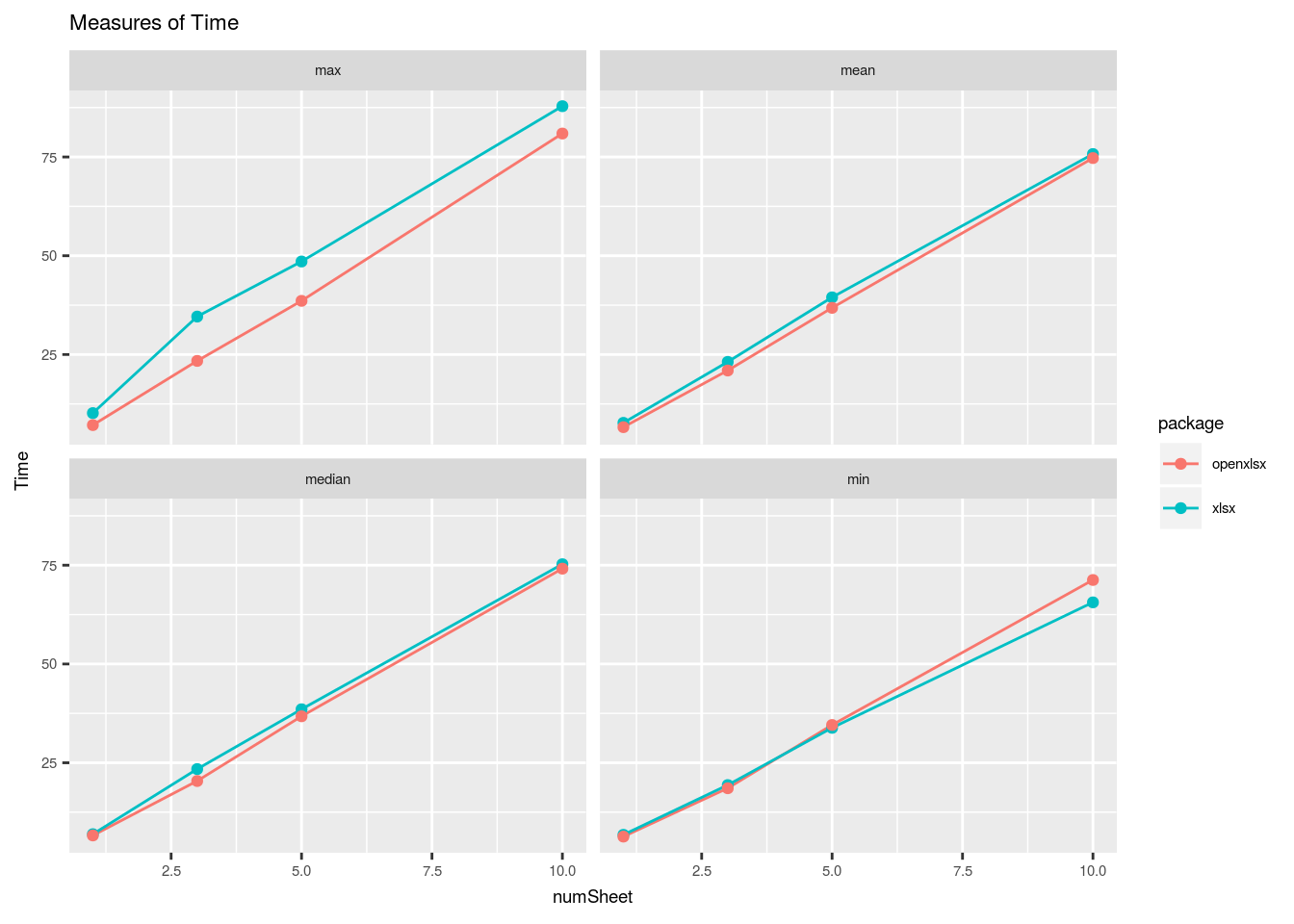Introduction

Saving your R dataframe to a .csv can be useful; being able to view the data all at once can help to see the bigger picture. Often though, multiple dataframes, all pieces of the same project, need to be viewed this way and related back to one another. In this case viewing becomes far easier when these dataframes are written to .xlsx across multiple sheets in a single workbook. Not to mention the time and energy saved when you no longer have to find and open multiple files.

Four packages in R are available to do just this. I generated some test data (a 30000 x 40 dataframe with sampled values between 1 and 100) and tested each one with varying levels of success.

# Set seed
set.seed(127)

# Create 1200000 sample values between 1 and 100 with replacement
sample.values <- sample(1:100, 1200000, replace = TRUE)

# Create 30000 x 40 matrix using sample values
sample.matrix <- as.data.frame(matrix(sample.values, nrow = 30000, ncol = 40))

# Character list to be used to name sheets in worksheet
num <- as.character(1:10)

Package use

The xlsx package

I ran into problems very early on with this package. The installation was dependent on the rJava package which gave an error during installation. Given that we’re using a Unix platform, the fix to this was to run sudo apt-get install r-cran-rjava in console. Executing this command succesfully installed the package and after restarting R-studio I was able to install and load the xlsx package.

The next problem I ran into was a java out of memory error, specifically java.lang.OutOfMemoryError. This time the fix was allocating more memory to Java trough the options(java.parameters = "-Xmx40000m") setting. The memory increase amount (and therefore the amount of data that can be written) is dependent on the amount of memory your computer can allocate to R. In this case I have allocated 40gig, which most of us do not have laying around. Once this was done I was able write up to 10 worksheets without any problems, but again, I ran into memory problems when I tried to scale up.

#---- Create function performing xlsx tasks ----#

Checkxlsx <- function ( num, sample.matrix ) {

wb <- xlsx::createWorkbook(type = "xlsx")

for ( i in 1:length(num) ) {

sheet.i = paste0("sheet", i)
sheet <- xlsx::createSheet(wb, sheet.i)
xlsx::addDataFrame(x = sample.matrix, sheet = sheet, col.names = FALSE, row.names = FALSE)
}

xlsx::saveWorkbook(wb, "xlsx_test.xlsx")
}

The XLConnect package

This package had all the same problems as the previous with one added extra. During testing, the writing process was done many times; each time it was done the previous .xlsx file saved as the same name needed to be deleted. If this wasn’t done R would continue attempting to write to the file indefinitely with no indications of stopping. There seems to be a fix for this using the createNames() function but it didn’t seem worth the effort.

#---- Create function performing XLConnect tasks ----#

CheckXLConnect <- function ( num, sample.matrix ) {

sapply(num, function(x) XLConnect::createSheet(wb, x))

for ( i in 1:length(num) ) {
XLConnect::writeWorksheet(wb, sample.matrix, as.character(i), header = FALSE)
}

XLConnect::saveWorkbook(wb)
}

The WriteXLS package

This package was easy enough to use with no apparent errors. The problem was that because this method writes the data directly into a workbook, instead of first creating it locally within the R environment, it was extremely slow.

# Name list of dataframes to be used to name sheets
names(sample.matrix.list) <- num

# Create list of dataframes
sample.matrix.list <- lapply(seq_len(as.numeric(num)), function(X) sample.matrix)

# Write list of dataframes to xlsx
WriteXLS(sample.matrix.list, "sample_matrix2.xlsx", col.names = FALSE)

The openxlsx package

Finally, I looked to the opnexlsx package. Again, no problems here; the most user friendly of the four.

#---- Create function performing openxlsx tasks ----#

CheckOpenxlsx <- function ( num, sample.matrix ) {

wb <- openxlsx::createWorkbook()

for ( i in 1:length(num) ) {
openxlsx::writeData(wb, i, sample.matrix, colNames = FALSE)
}

openxlsx::saveWorkbook(wb, file = "openxlsx_test.xlsx", overwrite = TRUE)
}

Microbenchmark test findings

After using each of the four packages I decided, taking usability, memory issues and speed into account, not to continue further with the WriteXLS and the XLConnect packages. I then tested the speed of the remaining two, openxlsx and xlsx, using the microbenchmark package with an evaluation number of 5 and varying sheet numbers.

1 Sheet with a 30000 x 40 dataframe run 5 times:

Unit: seconds
expr      min       lq     mean   median       uq       max neval
openxlsx 6.320148 6.367044 6.624763 6.610754 6.684871  7.140998     5
xlsx 6.770359 6.887633 7.706623 6.935831 7.756291 10.183000     5

3 Sheets with a 30000 x 40 dataframe run 5 times:

Unit: seconds
expr      min       lq     mean   median       uq      max neval
openxlsx 18.55911 19.72974 20.95868 20.39022 22.72171 23.39265     5
xlsx 19.36208 20.29261 23.14420 20.41937 21.03601 34.61094     5

5 Sheets with a 30000 x 40 dataframe run 5 times:

Unit: seconds
expr      min       lq     mean   median       uq      max neval
openxlsx 34.56846 36.61864 36.82423 36.77503 37.56867 38.59038     5
xlsx 33.85199 34.10490 39.47557 38.54957 42.31805 48.55332     5

10 Sheets with a 30000 x 40 dataframe run 5 times:

Unit: seconds
expr      min       lq     mean   median      uq      max neval
openxlsx 71.27145 72.24437 74.73020 74.14608 75.0495 80.93960     5
xlsx 65.60844 69.66743 75.74926 75.26078 80.3475 87.86212     5

Graphing the mean, median, min and max times for both the openxlsx and the xlsxpackages show that other than min time, the openxlsx package is faster.Conclusion

After testing each of the four packages using a dataframe of 30000 x 40 random numbers bewteen 1 and 100, I found that 2 of the packages, the XLConnectpackage and the WriteXLS package, were either not very user friendly or were simply too slow. The remaining two, the openxlsx package and the xlsx package, were then tested using the microbenchmark package with an evaluation number of 5 and various sheet numbers. The tests concluded that the openxlsx package was overall faster. With this in mind and with the ease of use for this package, it comes in as the favorite.